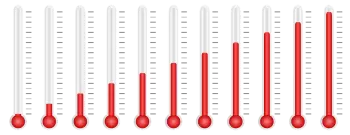# Celsius Scale and Degrees Centigrades with Conversion FormulasThe degree Celsius (its unit symbol is °C) is the unit of a temperature measurement scale, named after the Swedish astronomer Anders Celsius, who first proposed it in 1742. Some authors also use the name degree centigrade to refer to degree Celsius.

The Celsius scale is not an absolute scale. According to the second law, the absolute Celsius temperature scale is based on the existence of absolute 0º. This condition is only met by the Kelvin and Rankine scales.

The unit of temperature measurement in the international system of units is the kelvin. The degree Celsius belongs to the International System of Units as an accessory unit.

## How to Convert Temperature to Celsius Scale?

We can use the following formulas to convert the main temperature scales to the Celsius scale:

• The formula for degrees Fahrenheit to Celsius conversion °C = (5/9) × (°F - 32)

• Converting Kelvin to Celsius  °C = K - 273.15

We can use these temperature conversion formulas to convert degrees centigrade to degrees from other scales.

• Conversion from degrees Celsius to degrees Fahrenheit  °F = (9/5 × °C) + 32

• Conversion from Celsius to Kelvin à K = °C + 273.15

### For Example: 36 Degrees Celsius to Fahrenheit

Following the formula to convert Celsius to Fahrenheit:

(9/5 × 36ºF) + 32 = 96,8ºC

## What Is the Definition of the Celsius Scale?

The Celsius scale is defined as the temperature scale where 0 is the freezing point of water, and the boiling point of water is 100 degrees Celsius in the standard atmosphere.

In the original scale, these values ​​were inverted: they were zero for the boiling point and one hundred for the melting point of water. However, in 1745 Jean-Pierre Christin and Carlos Linnaeus proposed reversing the scale, transforming it into the commonly used one.

The current official definition of the Celsius scale places 0.01°C as the triple point of water and one degree as 1/273.16 of the temperature difference between the triple point of water and absolute zero. In short, the triple point of water is defined as being precisely 273.14 K and 0.01 °C.

This definition does three things:

1. It fixes the magnitude of the kelvin unit as being precisely 1 part in 273.16 parts, the difference between absolute zero and the triple point of water.

2. It turns out that one kelvin has the same magnitude as a one-degree increment on the Celsius scale.

3. It establishes the difference between the two scales' null points as being precisely 273.15 kelvins (0 K = −273.15 °C and 273.16 K = 0.01 °C).

## What Are the Common Uses of the Celsius Scale?

The Celsius scale is widely used to express the temperatures of daily use, from the temperature of the air to that of a host of domestic devices (ovens, fryers, hot water, refrigeration, etc.).

It is also used in scientific and technological work, although the use of the Kelvin scale is required in many cases. For this reason, it is common to convert Fahrenheit to centigrade degrees or kelvins.

The Celsius scale is used in most parts of the world daily. However, the Fahrenheit scale is used more frequently in some states, especially the Anglo-Saxons.

## What Are the Reference Points in a Thermometer with a Celsius Scale?

The Celsius scale has two essential reference points:

• The first is the melting point of ice (or the freezing point of water), set as 0 °C.

• The second one is the boiling point of water, defined as 100 degrees centigrades.

Once we have purified it, the melting point of ice is a relatively exact specification. However, the boiling point of water is less precise due to its dependency on atmospheric pressure.

In this range, any increase of heat energy to any amount of matter entails increasing its temperature.

## What Is the Difference Between Degrees Celsius and Degrees Centigrade?

Since there are one hundred divisions between these two reference points, the original term for this system was degree centigrade. In 1948, the 9th Conférence générale des poids et mesures (CR 64) officially changed the name to degree Celsius to remove some ambiguities.

The decision of the Conférence générale des poids et mesures was aimed at eliminating ambiguities and unequivocally associating the creation of the scale with Celsius. In addition, the scale was standardized to those of Kelvin (raised by lord Kelvin), Fahrenheit, Réaumur, and Rankine, all of them with the name of their inventor.

Despite this, people often refer to degrees centigrade as "degrees Celsius," in the same way that they use the concept of centigrade scale.

## Who Invented the Celsius Scale of Temperature?

The creator of the Celsius scale was Anders Celsius (1701-1744), a scientist of Swedish origin. Born in the Swedish Empire,  he was a professor of astronomy at the University of Uppsala. He supervised the construction of its observatory, in charge of which he was from 1740.

He was interested in observing the northern lights and measuring the planet's flattening at its poles. However, his best-known scientific contribution was the creation of the temperature scale that bears his name, which he proposed to the Swedish Academy of Sciences. as a replacement for the Fahrenheit scale of German origin.

He died in 1744 being a victim of tuberculosis. However, he enjoyed numerous awards in the scientific area during his lifetime, such as his acceptance into the Royal Society, the Leopoldina Academy of Natural Sciences, or the Prussian Academy of Sciences. Later, one of the craters on the Moon was named in his honor.

Author:

Published: October 25, 2018
Last review: May 20, 2022